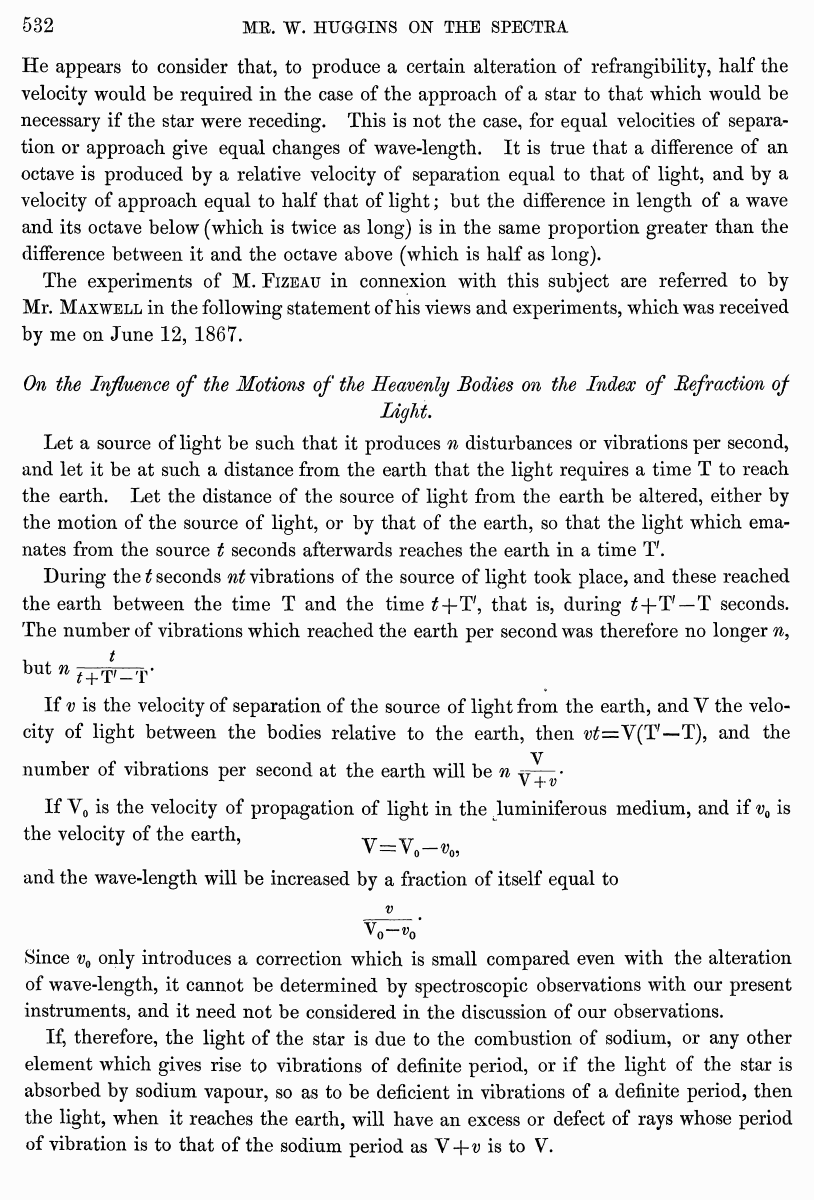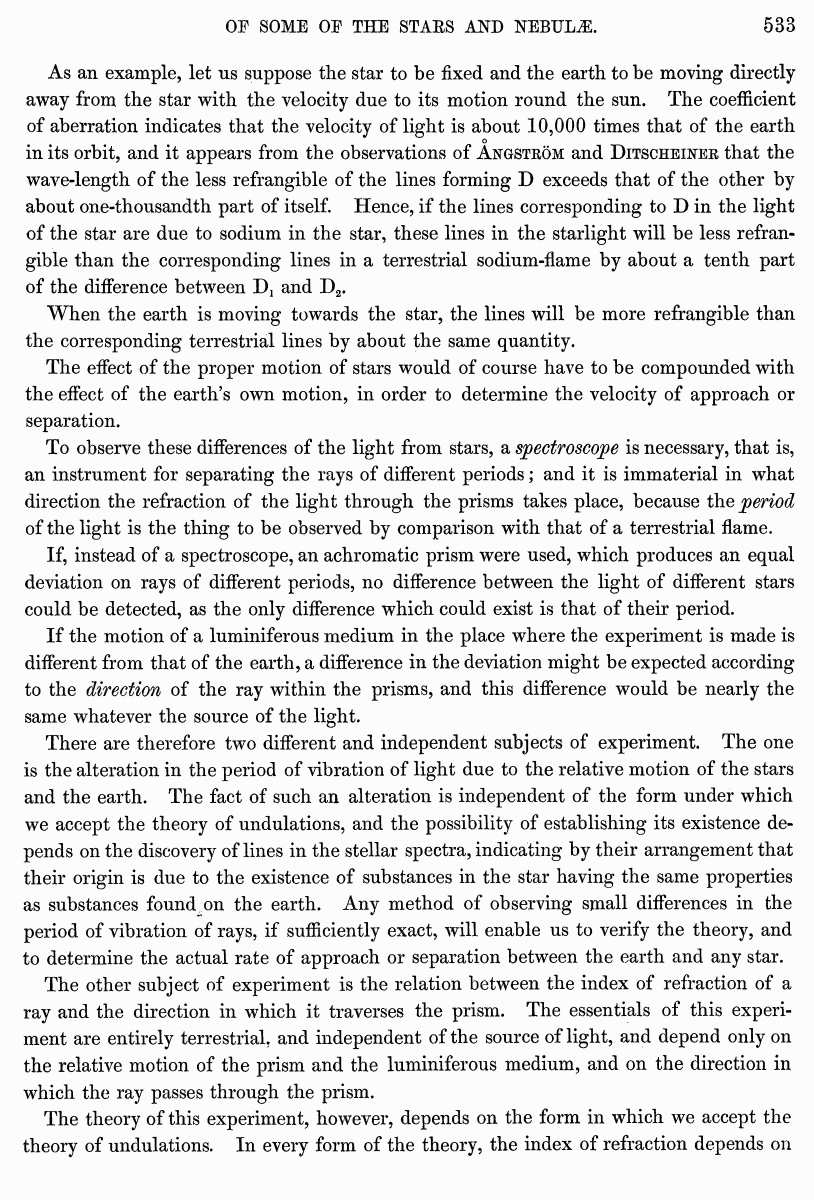# Huggins, Maxwell, 1868 //Philosophical Transactions of the Royal Society of London 158 (1868)

В начало   Другие форматы   <<<     Страница 532   >>>529  530  531  532 533  534  535  536  537  538  539  540  541  542  543  544  545  546  547  548  549  550  551  552  553  554  555  556  557  558  559  560  561  562  563  564 He appears to consider that, to produce a certain alteration of refrangibility, half the velocity would be required in the case of the approach of a star to that which would be necessary if the star were receding. This is not the case, for equal velocities of separation or approach give equal changes of wave-length. It is true that a difference of an octave is produced by a relative velocity of separation equal to that of light, and by a velocity of approach equal to half that of light; but the difference in length of a wave and its octave below (which is twice as long) is in the same proportion greater than the difference between it and the octave above (which is half as long). The experiments of M. Fizeau in connexion with this subject are referred to by Mr. Maxwell in the following statement of his views and experiments, which was received by me on June 12, 1867. On the Influence of the Motions of the Heavenly Bodies on the Index of Refraction of Light. Let a source of light be such that it produces n disturbances or vibrations per second, and let it be at such a distance from the earth that the light requires a time T to reach the earth. Let the distance of the source of light from the earth be altered, either by the motion of the source of light, or by that of the earth, so that the light which emanates from the source t seconds afterwards reaches the earth in a time T'. During the t seconds nt vibrations of the source of light took place, and these reached the earth between the time T and the time tf+T', that is, during tf+T'—T seconds. The number of vibrations which reached the earth per second was therefore no longer n, but n ; + T*,_T- If v is the velocity of separation of the source of light from the earth, and V the velocity of light between the bodies relative to the earth, then ^=Y(T'—T), and the number of vibrations per second at the earth will be n ^r— ■ X V + w If Y0 is the velocity of propagation of light in the .luminiferous medium, and if v0 is the velocity of the earth, V—v V - V0 W0, and the wave-length will be increased by a fraction of itself equal to v Vo-vo Since v„ only introduces a correction which is small compared even with the alteration of wave-length, it cannot be determined by spectroscopic observations with our present instruments, and it need not be considered in the discussion of our observations. If, therefore, the light of the star is due to the combustion of sodium, or any other element which gives rise to vibrations of definite period, or if the light of the star is absorbed by sodium vapour, so as to be deficient in vibrations of a definite period, then the light, when it reaches the earth, will have an excess or defect of rays whose period of vibration is to that of the sodium period as V -\-v is to V. As an example, let us suppose the star to be fixed and the earth to be moving directly away from the star with the velocity due to its motion round the sun. The coefficient of aberration indicates that the velocity of light is about 10,000 times that of the earth o in its orbit, and it appears from the observations of Angstrom and Ditscheiner that the wave-length of the less refrangible of the lines forming D exceeds that of the other by about one-thousandth part of itself. Hence, if the lines corresponding to D in the light of the star are due to sodium in the star, these lines in the starlight will be less refrangible than the corresponding lines in a terrestrial sodium-flame by about a tenth part of the difference between D, and D2. When the earth is moving towards the star, the lines will be more refrangible than the corresponding terrestrial lines by about the same quantity. The effect of the proper motion of stars would of course have to be compounded with the effect of the earth’s own motion, in order to determine the velocity of approach or separation. To observe these differences of the light from stars, a spectroscope is necessary, that is, an instrument for separating the rays of different periods; and it is immaterial in what direction the refraction of the light through the prisms takes place, because the period of the light is the thing to be observed by comparison with that of a terrestrial flame. If, instead of a spectroscope, an achromatic prism were used, which produces an equal deviation on rays of different periods, no difference between the light of different stars could be detected, as the only difference which could exist is that of their period. If the motion of a luminiferous medium in the place where the experiment is made is different from that of the earth, a difference in the deviation might be expected according to the direction of the ray within the prisms, and this difference would be nearly the same whatever the source of the light. There are therefore two different and independent subjects of experiment. The one is the alteration in the period of vibration of light due to the relative motion of the stars and the earth. The fact of such an alteration is independent of the form under which we accept the theory of undulations, and the possibility of establishing its existence depends on the discovery of lines in the stellar spectra, indicating by their arrangement that their origin is due to the existence of substances in the star having the same properties as substances found on the earth. Any method of observing small differences in the period of vibration of rays, if sufficiently exact, will enable us to verify the theory, and to determine the actual rate of approach or separation between the earth and any star. The other subject of experiment is the relation between the index of refraction of a ray and the direction in which it traverses the prism. The essentials of this experiment are entirely terrestrial, and independent of the source of light, and depend only on the relative motion of the prism and the luminiferous medium, and on the direction in which the ray passes through the prism. The theory of this experiment, however, depends on the form in which we accept the theory of undulations. In every form of the theory, the index of refraction depends on